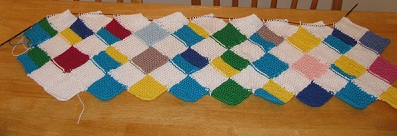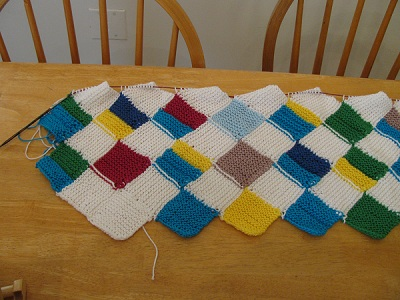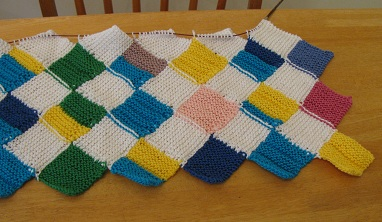## Prime Factorization Blanket – to 29

I got done another row of numbers on the Prime Factorization Blanket for my arriving niece!It’s hard to see the ridges in the solid colors, so here are close-ups of the left half, then right half:The bottom row in the picture is 1 (white), 2 (blue), 3 (yellow), 4 = 2 x 2, 5 (green).

The second row is 10 = 2 x 5, 11 (red), 12 = 2 x 2 x 3, 13 (tan), 14 = 2 x 7, 15 = 3 x 5.

The top row is 20 = 2 x 2 x 5, 21 = 3 x 7, 22 = 2 x 11, 23 (baby blue), 24 = 2 x 2 x 2 x 3, 25 = 5 x 5.

Now the right half:Here we have the bottom row of 5 (green), 6 = 2 x 3, 7 (dark purple), 8 = 2 x 2 x 2, 9 = 3 x 3

The second row is 15 = 3 x 5, 16 = 2 x 2 x 2 x 2, 17 (pink), 18 = 2 x 3 x 3, 19 (dark pink).

The third row is 25 = 5 x 5, 26 = 2 x 13, 27 = 3 x 3 x 3, 28 = 2 x 2 x 7, 29 (periwinkle)

I really like the way it’s turning out!

You can read more about my prime factorization knitting in previous blog posts or via my Pinterest board. And don’t forget to look in my cafe press shop for prime factorization t-shirts.

My posts on Mathematical Knitting and related topics are now gathered at Sonderknitting.